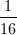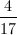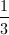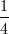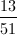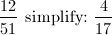## Example Questions

### Example Question #139 : Statistics

A bag contains four blue marbles, eight red marbles, and six orange marbles. If a marble is randomly selected from the bag, what is the probability that the marble will be either red or orange?

2/5

5/9

1/3

7/9

2/3

7/9

Explanation:

The probability of an event is the ratio of the number of desired outcomes to the total number of possible outcomes. In this problem, the total number of outcomes is equal to the total number of marbles in the bag. There are four blue, eight red, and six orange marbles, so the total number of marbles is the sum of four, eight, and six, or eighteen.

We are asked to find the probability of choosing a marble that is either red or orange. This means we have to consider the number of marbles that are either red or orange. Because there are eight red and six orange, there are fourteen marbles that are either red or orange.

The probability is thus fourteen out of eighteen, because there are fourteen red or orange marbles, out of a total of eighteen marbles. We will need to simplify the fraction 14/18.

Probability = 14/18 = 7/9

### Example Question #140 : Statistics

Two dice are rolled. Find the probability that the numbers sum to 4.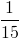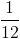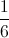Explanation:

The possible dice combinations that sum to 4 are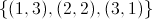.

The number of all possible dice combinations is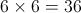. (6 numbers on each of the two dice.)

So the probability that the numbers sum to 4  =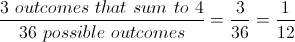### Example Question #41 : How To Find The Probability Of An Outcome

Jackie is a contestant on a gameshow. She has to pick marbles out of a big bag to win various amounts of money. The bag contains a total of 200 marbles.

There are 100 red marbles worth $10 each, 50 blue marbles worth$20 each, 30 green marbles worth $50 each, 15 white marbles worth$100 each and 5 black marbles worth $1000 each. If she picks once, what percent chance does she have of picking a$1000 marble?

5%

.05%

3%

2.5%

2.5%

Explanation:

There are 5 black marbles worth \$1000 dollars out of the total of 200 marbles.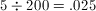or 2.5%

### Example Question #42 : How To Find The Probability Of An Outcome

What is the probability that you will pull out 3 diamonds in a standard deck without replacement.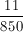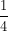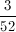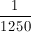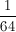Explanation:

There are 13 diamonds in a standard deck of 52 cards. So, you have a 13/52 (1/4) chance of getting a diamond; then a 12/51 (4/17) chance of pulling the next diamond; last, there is a 11/50 chance of getting the third diamond. When you combine probabilities, you multiply the individual probabilities together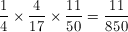### Example Question #43 : How To Find The Probability Of An Outcome

On Halloween there is a bowl of candy.  33 pieces are gum drops, 24 are candy corn, 15 are suckers and 28 are hard candies.  Without looking in the bowl, what are the chances that you pull a gum drop?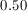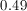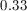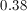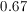Explanation:

Probability = # Gum Drops / # Total Number of Candies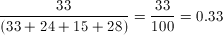### Example Question #44 : How To Find The Probability Of An Outcome

What is the probability of pulling out 4 diamonds in a standard deck of cards, without replacement?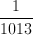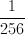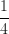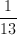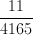Explanation:

There are 52 cards in a standard deck; 13 of them are diamonds. This means that there is a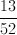chance that the first card you pick will be a diamond. The probability of pulling another diamond as your next card is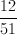; next diamond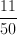; last diamond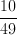.

In order to get one probability, mulitply the individual probabilities together.

### Example Question #41 : Probability

A coporation is deciding who should sit on its leadership committee. If twenty people have applied, and the corporation will choose six applicants to sit on the committee, how many committees are possible?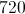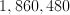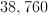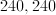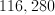Explanation:

This question requires us to make use of the combination formula. In general, if we have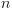total objects or things to choose from, the number of different groups of sizethat we can make is equal to the following: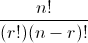where the ! symbol denotes a factorial. In general,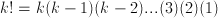.

For example,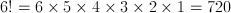.

We have 20 people from whom to select, and each group is going to have 6 people.  Therefore, we will let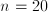and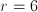.

number of combinations =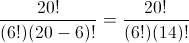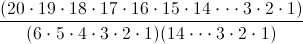=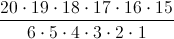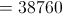The answer is.

### Example Question #46 : How To Find The Probability Of An Outcome

What is the probability that two dice rolled will add up to 5?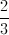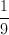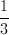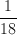Explanation:

There are 6 probabilities for each dice and there are 2 dice. So, you would multiply the individual probabilities together and get 36 possible outcomes.

In order for 2 dice to add to a sum of 5, you would need to roll:

1 4; 2 3; 3 2; 4 1 [each dice can roll as either number]

That is a total of 4 outcomes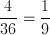### Example Question #47 : How To Find The Probability Of An Outcome

A bag containing only red, green and blue marbles has 10 red, 20 green, and 20 blue marbles.  If Laura reaches in and removes a single marble at random, what is the probability she draws a green marble?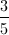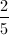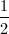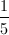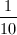Explanation:

Probability = the number of desired outcomes divided by the number of total possible outsomes.  20 marbles are green out of a total of 50.  Therefore: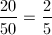### Example Question #48 : How To Find The Probability Of An Outcome

A deck of 52 cards is divided equally into four suits: hearts, diamonds, clubs and spades, and two cards are drawn at random.  The first card drawn is a heart. What is the probability that the second card drawn is also a heart?